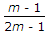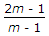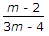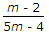# Mechanical Engineering - Strength of Materials

Exercise : Strength of Materials - Section 1
16.
The bending moment at a point on a beam is the algebraic __________ of all the moments on either side of the point.
sum
difference
Explanation:
No answer description is available. Let's discuss.

17.
The maximum diameter of the hole that can be punched from a plate of maximum shear stress 1/4th of its maximum crushing stress of punch, is equal to (where t = Thickness of the plate)
t
2t
4t
8t
Explanation:
No answer description is available. Let's discuss.

18.
Two closely coiled helical springs 'A' and 'B' are equal in all respects but the number of turns of spring 'A' is half that of spring 'B' The ratio of deflections in spring 'A' to spring 'B' is
1/8
1/4
1/2
2
Explanation:
No answer description is available. Let's discuss.

19.
The deformation per unit length is called
tensile stress
compressive stress
shear stress
strain